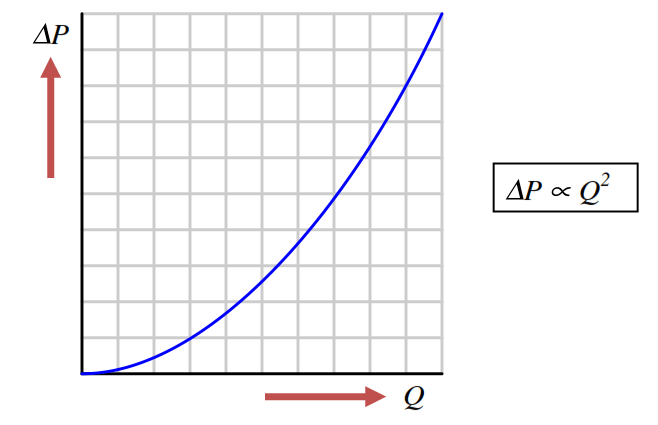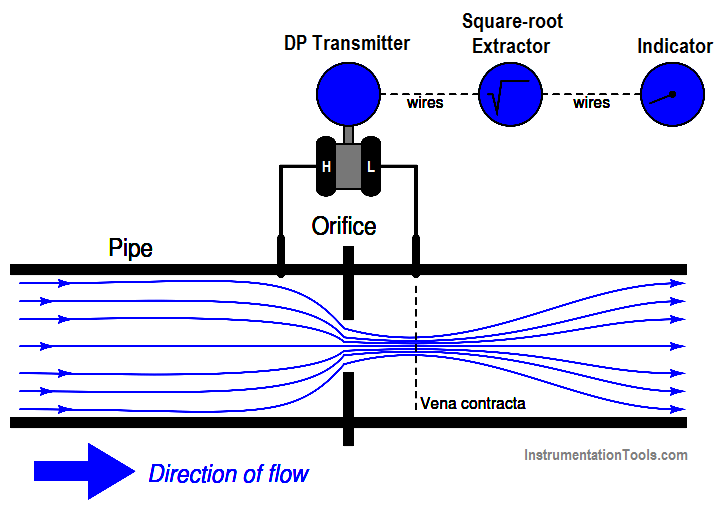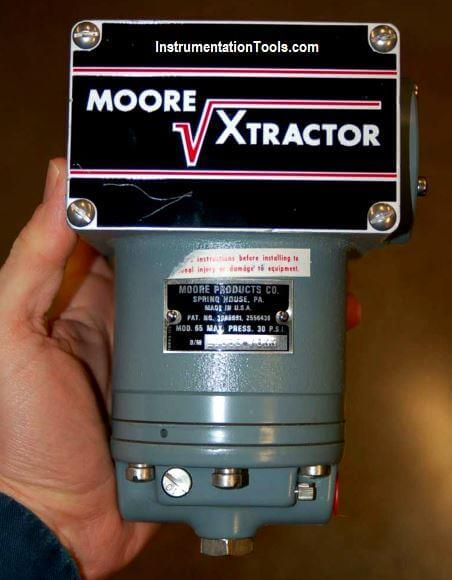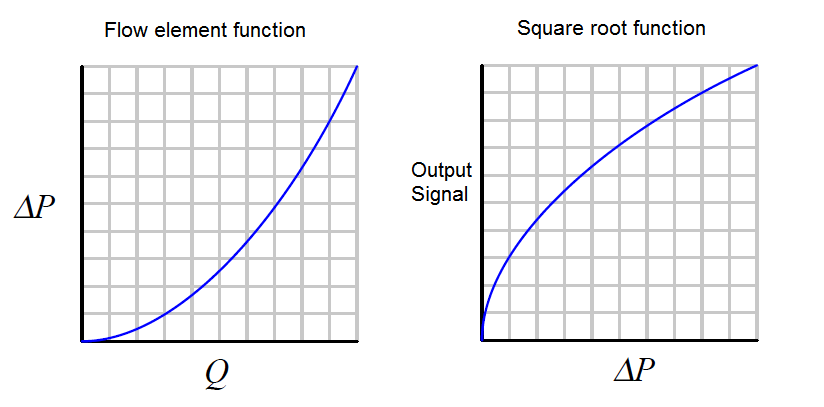# What is Square Root Extractor?

The relationship between ﬂow rate and diﬀerential pressure for any ﬂuid-accelerating ﬂow element is non-linear. When plotted on a graph, the relationship between ﬂow rate (Q) and diﬀerential pressure (∆P) is quadratic, like one-half of a parabola.

Diﬀerential pressure developed by a venturi, oriﬁce plate, pitot tube, or any other acceleration-based ﬂow element is proportional to the square of the ﬂow rate:

## Relationship of Flow rate and Diﬀerential pressureThe traditional means of implementing the necessary signal characterization was to install a “square root” function relay between the transmitter and the ﬂow indicator, as shown in the following diagram:## Square Root Extractor

In the days of pneumatic instrumentation, this square-root function was performed in a separate device called a square root extractor. The Foxboro model 557 (2) and Moore Products model 65 (1) pneumatic square root extractors are classic examples of this technology :### Internal Parts of Square-root ExtractorPneumatic square root extraction relays provide a square-root function that the relays were able to serve their purpose in characterizing the output flow signal of a pressure sensor to yield a signal representing ﬂow rate.

The following table shows the ideal response of a pneumatic square root relay:As you can see from the table, the square-root relationship is most evident in comparing the input and output percentage values. For example, at an input signal pressure of 6 PSI (25%), the output signal percentage will be the square root of 25%, which is 50% (0.5 = √0.25) or 9 PSI as a pneumatic signal.

At an input signal pressure of 10 PSI (58.33%), the output signal percentage will be 76.38%, because of 0.7638 = √0.5833, yielding an output signal pressure of 12.17 PSI.

When graphed, the function of a square-root extractor is precisely opposite (inverted) of the quadratic function of a flow-sensing element such as an orifice plate, venturi, or pitot tube:When we connect the output of the DP transmitter to the input of Square Root Extractor, the square-root function applies to the output signal – the result is an output signal that tracks linearly with flow rate (Q). An instrument connected to the square root relay’s signal will, therefore, register the flow rate as it should.

Note: We are not using the square-root extractor devices anymore, these are replaced with modern DP Transmitters.

The modern solution to this problem is to incorporate square-root signal characterization either inside the transmitter or inside the receiving instrument (e.g. indicator, recorder, or controller). Either way, the square-root function must be implemented somewhere in the loop in order that ﬂow may be accurately measured throughout the operating range.

Author: Tony R. Kuphaldt